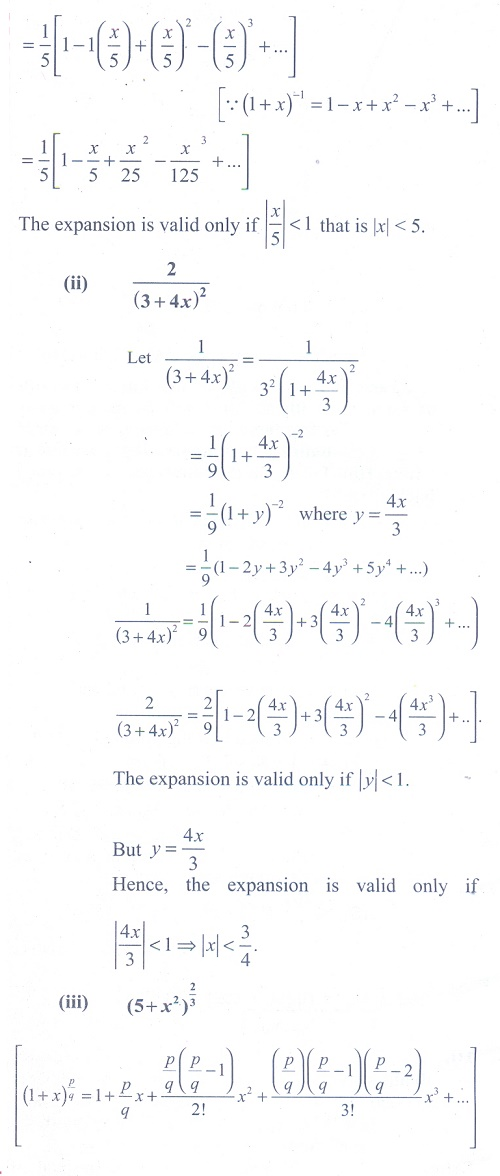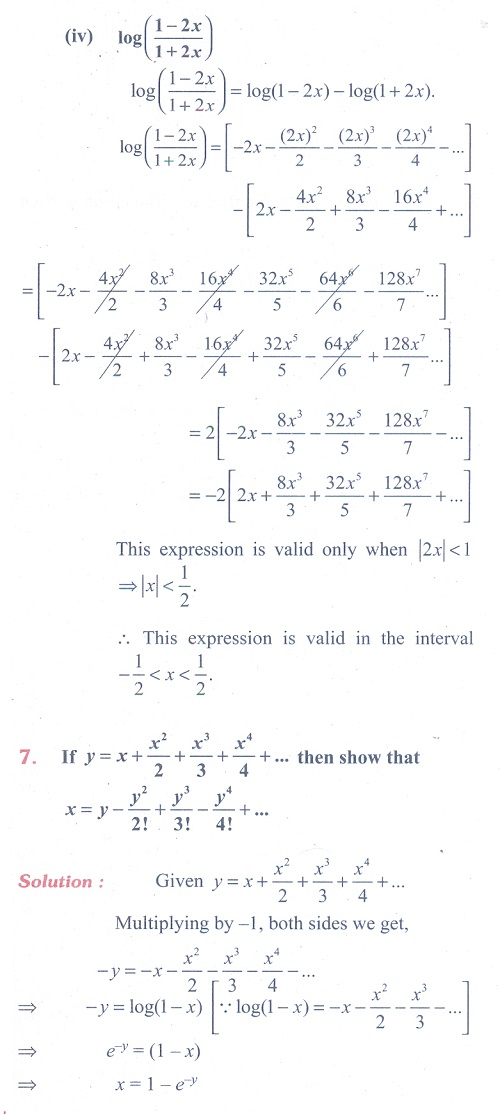Home | | Maths 11th std | Exercise 5.4: Infinite Sequences and Series

# Exercise 5.4: Infinite Sequences and Series

Maths Book back answers and solution for Exercise questions - Mathematics : Binomial Theorem, Sequences and Series: Infinite Sequences and SeriesTags : Problem Questions with Answer, Solution | Mathematics , 11th Mathematics : UNIT 5 : Binomial Theorem, Sequences and Series
Study Material, Lecturing Notes, Assignment, Reference, Wiki description explanation, brief detail
11th Mathematics : UNIT 5 : Binomial Theorem, Sequences and Series : Exercise 5.4: Infinite Sequences and Series | Problem Questions with Answer, Solution | Mathematics